# Deep learning and Dogs vs. Cats - Part 1½

## Data set https://www.kaggle.com/c/dogs-vs-cats¶

(the data set should be installed in two directories just above the one where this notebook is located: train and test1)

### http://ricardodeazambuja.com/deep_learning/2017/02/10/easy-peasy_deep_learning_one_and_a_half/¶

In :
# The package 'os' will be necessary to find the current
# working directory os.getcwd() and also to list all
# files in a directory os.listdir().
import os

# https://docs.python.org/2/library/multiprocessing.html
from multiprocessing import Pool

# Only used for the final test
import urllib, cStringIO

# Yep, I don't like to import numpy as np :P
import numpy

# As I've explained somewhere, Scipy will help us
# reading (using Pillow) and resizing images.
import scipy.misc

# Pyplot is what we use for plotting
import matplotlib.pyplot as plt

# And here is the Keras stuff:

# We don't really need this since it would
# be easy do code something like this ourselves
from keras.utils import np_utils

# https://keras.io/getting-started/sequential-model-guide/
from keras.models import Sequential
from keras.models import Model

from keras.layers import Dense
from keras.layers import Activation
from keras.layers import Dropout

# https://keras.io/optimizers/
from keras.optimizers import SGD

# While the method for saving a model is
# part of the model object, to load we need
# to import something else


## 1) Load the data sets¶

In :
# Returns the current working directory
# (where the Python interpreter was called)
path = os.getcwd()

# The path separator used by the OS:
sep = os.path.sep

# This is the directory where the training images are located
dirname = "train"

# Generates a list of all images (actualy the filenames) from the training set,
# but it will also include the full path
imagePaths = [path+sep+dirname+sep+filename
for filename in os.listdir(path+sep+dirname)]

In :
# To speed up things, I will use parallel computing!
# Pool lets me use its map method and select the number of parallel processes.

def import_training_set(args):
'''
Reads the image from imagePath, resizes
and returns it together with its label and
original index.

'''
index,imagePath,new_image_size = args

# Split will literally split a string at that character
# returning a list of strings.
# First we split according to the os.path.sep and keep
# only the last item from the list gererated ([-1]).
# This will give us the filename.
filename = imagePath.split(os.path.sep)[-1]

# Then we split it again using "."
# and extract the first item ():
label = filename.split(".")

# and the second item ():
original_index = filename.split(".")

# Resizes the image (downsampling) to new_image_size and
# converts it to a 1D list (flatten).
input_vector = scipy.misc.imresize(image,new_image_size).flatten()

return (index,(original_index,label,input_vector))

In :
%%time
# The size of the resized image.
# After we apply the flatten method, it will become a list of 32x32x3 items (1024x3).
# (Where is the 'x3' coming from? Our images are composed of three colors!)
new_image_size = 32,32

number_of_parallel_processes = 7

# When the map is finished, we will receive a list with tuples:
# (index,(original_index,label,input_vector)) => (index,(original_index,'category',1D resized image))
#
# There's no guarantee about the ordering because they are running in parallel.
ans = Pool(number_of_parallel_processes).map(import_training_set,[(i,img,new_image_size)
for i,img in enumerate(imagePaths)])

CPU times: user 236 ms, sys: 99.4 ms, total: 336 ms
Wall time: 40.2 s

In :
# Because import_training_set returns a tuple like this:
# (index,(original_index,label,input_vector))
# and index is unique, we can convert to a dictionary
# to solve our problem with unordered items:
training_set = dict(ans)

# Gives a hint to the garbage collector...
del ans

In :
# Let's verify if we loaded all images:
len(training_set)

Out:
25000
In :
# And now test to see what is inside:
training_set,training_set

Out:
(('0', 'cat', array([211, 172,  94, ...,   3,   3,   0], dtype=uint8)),
('9999', 'dog', array([249, 243, 235, ..., 254, 254, 254], dtype=uint8)))

## 2) Prepare the data set for Keras¶

In :
# Let's imagine we don't know how many different labels we have.
# (we know, they are 'cat' and 'dog'...)
# A Python set can help us to create a list of unique labels.
# (i=>filename index, i=>label, i=>1D vector)
unique_labels = set([i for i in training_set.values()])

# With a list of unique labels, we will generate a dictionary
# to convert from a label (string) to a index (integer):
labels2int = {j:i for i,j in enumerate(unique_labels)}

# Creates a list with labels (ordered according to the dictionary index)
labels = [labels2int[i] for i in training_set.values()]

In :
# Instead of '1' and '0', the function below will transform our labels
# into vectors [0.,1.] and [1.,0.]:
labels = np_utils.to_categorical(labels, 2)

In :
# First, we will create a numpy array going from zero to len(training_set):
# (dtype=int guarantees they are integers)
random_selection = numpy.arange(len(training_set),dtype=int)

# Then we create a random state object with our seed:
# (the seed is useful to be able to reproduce our experiment later)
seed = 12345
rnd = numpy.random.RandomState(seed)

# Finally we shuffle the random_selection array:
rnd.shuffle(random_selection)

In :
test_size=0.25 # we will use 25% for testing purposes

# Breaking the code below to make it easier to understand:
# => training_set[i][-1]
# Returns the 1D vector from item 'i'.
# => training_set[i][-1]/255.0
# Normalizes the input values from 0. to 1.
# => random_selection[:int(len(training_set)*(1-test_size))]
# Gives us the first (1-test_size)*100% of the shuffled items
trainData = [training_set[i][-1]/255.0 for i in random_selection[:int(len(training_set)*(1-test_size))]]
trainData = numpy.array(trainData)

trainLabels = [labels[i] for i in random_selection[:int(len(training_set)*(1-test_size))]]
trainLabels = numpy.array(trainLabels)

testData = [training_set[i][-1]/255.0 for i in random_selection[:int(len(training_set)*(test_size))]]
testData = numpy.array(testData)

testLabels = [labels[i] for i in random_selection[:int(len(training_set)*(test_size))]]
testLabels = numpy.array(testLabels)


### Before we move forward, let's create a super simple 2x2 RGB image and try to understand what are we doing with our images before injecting them into the network:¶

In :
# Using sympy to print nice LaTeX based matrices:
# http://docs.sympy.org/dev/tutorial/printing.html
from sympy import Matrix, init_printing
# init_printing(use_latex=True)
init_printing(use_latex='png')  # Makes easier to add to a post without using MathML

In :
img = numpy.zeros((2,2,3))  # RGB=>3
img[0,0,0] = 1.  #(0,0) - R layer=>0
img[0,1,1] = 1.  #(0,1) - G layer=>1
img[1,0,2] = 1.  #(0,0) - B layer=>1
img[1,1,:] = 0.  #(1,1) - R,G,B layers=>0. In RGB, a zero value for all colours means black.
img

Out:
array([[[ 1.,  0.,  0.],
[ 0.,  1.,  0.]],

[[ 0.,  0.,  1.],
[ 0.,  0.,  0.]]])
In :
plt.imshow(img,interpolation='none')
plt.show()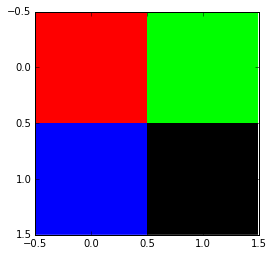### Now, we verify what flatten is doing to our image:¶

In :
# First, visualize the R,G and B matrices using Sympy / LaTeX:
Matrix(img[:,:,0]) , Matrix(img[:,:,1]), Matrix(img[:,:,2])

Out: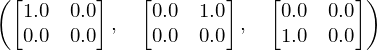In :
print "Flattened (now 1D array):"
print img.flatten()
print
print "Flattened (now highlighting how the 2x2 matrices become a 1D array):"
print "(Flatten gets element 0 from all 3 matrices, then element 1... and at the end just glue them together)"
print img.flatten().reshape(4,3)
print
print "Original (2x2x3 array):"
print img.flatten().reshape((2,2,3))

Flattened (now 1D array):
[ 1.  0.  0.  0.  1.  0.  0.  0.  1.  0.  0.  0.]

Flattened (now highlighting how the 2x2 matrices become a 1D array):
(Flatten gets element 0 from all 3 matrices, then element 1... and at the end just glue them together)
[[ 1.  0.  0.]
[ 0.  1.  0.]
[ 0.  0.  1.]
[ 0.  0.  0.]]

Original (2x2x3 array):
[[[ 1.  0.  0.]
[ 0.  1.  0.]]

[[ 0.  0.  1.]
[ 0.  0.  0.]]]

In :
(Matrix(img[:,:,0]) , Matrix(img[:,:,1]), Matrix(img[:,:,2])),Matrix(img.flatten().reshape(4,3).T),Matrix(img.flatten())

Out: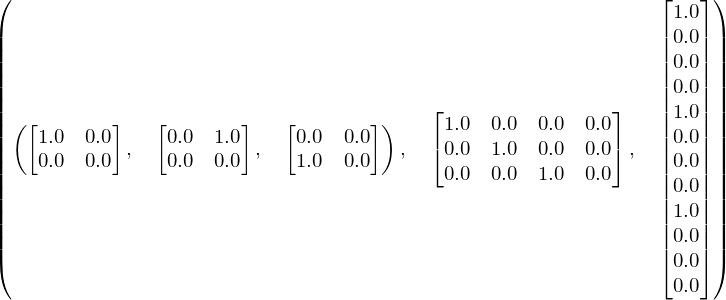### If you reshape an image, the wrong way, it can look very crazy!¶

In :
plt.figure(figsize=(20,10))
plt.subplot(141)
plt.imshow((testData.reshape(32,32,3)), interpolation='none')
plt.title("Original")
plt.subplot(142)
plt.imshow((numpy.rollaxis(testData.reshape(32,32,3), 1, 0)).reshape(32,32,3), interpolation='none')
plt.title("Crazy 1")
plt.subplot(143)
plt.imshow((numpy.rollaxis(testData.reshape(32,32,3), 2, 0)).reshape(32,32,3), interpolation='none')
plt.title("Crazy 2")
plt.subplot(144)
plt.imshow(numpy.rollaxis(testData.reshape(3,32,32), 0, 3), interpolation='none')
plt.title("Crazy 3")
plt.show()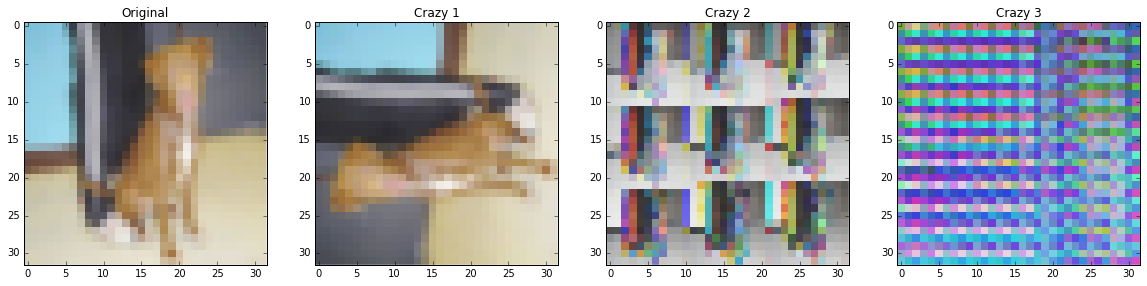In :
# # I'm not using it, but it's useful, so I will let it here ;)
# old_options = numpy.get_printoptions()
# # numpy.set_printoptions(old_options)
# old_options

In :
# The original image RGB matrices
img_round = numpy.round(testData.reshape(32,32,3),decimals=2)
Matrix(img_round[:,:,0]),Matrix(img_round[:,:,1]),Matrix(img_round[:,:,2])

Out: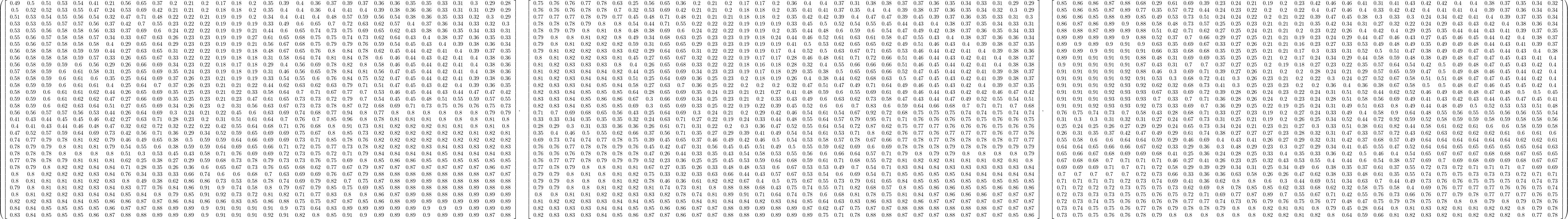## 3) Create a Sequential model¶

Now, we will give names to our layers and make life easier.

In :
# https://keras.io/getting-started/sequential-model-guide/
# define the architecture of the network
model_rnd = Sequential()

# input layer has size "input_dim" (new_image_size*new_image_size*3).
# The first hidden layer will have size 768, followed by 384 and 2.
# 3072=>768=>384=>2
input_len = new_image_size*new_image_size*3

# A Dense layer is a fully connected NN layer (feedforward)
# https://keras.io/layers/core/#dense
# init="uniform" will initialize the weights / bias randomly

# https://keras.io/layers/core/#activation
# https://keras.io/activations/

# Now this layer will have output dimension of 384

# Because we want to classify between only two classes (binary), the final output is 2


### But the old model was created without names!¶

We have, at least, two options:

1. find or change the names
2. recover the weights and load into the new network
In :
my_97perc_acc = load_model('my_97perc_acc.h5')

In :
score = my_97perc_acc.evaluate(testData, testLabels, batch_size=128, verbose=0)
print('Test score:', score)
print('Test accuracy:', score)

('Test score:', 0.13020923321247102)
('Test accuracy:', 0.97744000049591062)

In :
# layers is a list with Keras layers (instances)
my_97perc_acc.layers

Out:
[<keras.layers.core.Dense at 0x146804350>,
<keras.layers.core.Activation at 0x14394c510>,
<keras.layers.core.Dense at 0x10f61de10>,
<keras.layers.core.Activation at 0x141e62950>,
<keras.layers.core.Dense at 0x14394c550>,
<keras.layers.core.Activation at 0x141a1b750>]
In :
# Just to make life easier
first_layer = my_97perc_acc.layers
first_layer_after_activation = my_97perc_acc.layers
second_layer = my_97perc_acc.layers
second_layer_after_activation = my_97perc_acc.layers
classifier_layer = my_97perc_acc.layers


### 1. Find/change the layer names¶

In :
# Keras automatically attributed layer names
for layer in my_97perc_acc.layers:
print layer.name

dense_1
activation_1
dense_2
activation_2
dense_3
activation_3

In :
# But I think it's nicer to change the names to match the new model
first_layer.name = "Input_layer"
first_layer_after_activation.name = "Input_layer_act"
second_layer.name = "Hidden_layer"
second_layer_after_activation.name = "Hidden_layer_act"

In :
# https://keras.io/getting-started/faq/#how-can-i-obtain-the-output-of-an-intermediate-layer

model = model_rnd

# model = my_97perc_acc

layer_name = 'Input_layer_act'
input_layer_out = Model(input=model.input, output=model.get_layer(layer_name).output)

layer_name = 'Hidden_layer_act'
hidden_layer_out = Model(input=model.input, output=model.get_layer(layer_name).output)

In :
idx = 1
input_image = testData[idx]
first_output = input_layer_out.predict(input_image.reshape(1,testData[idx].shape))
second_output = hidden_layer_out.predict(input_image.reshape(1,testData[idx].shape))
second_output.resize(11*11*3)
final_output =  model.predict(input_image.reshape(1,input_image.shape))

In :
plt.figure(figsize=(20,10))
plt.subplot(131)
plt.imshow((input_image.reshape(32,32,3)), interpolation='none')
plt.title("Original - "+str(final_output))
plt.subplot(132)
plt.imshow(first_output.reshape(16,16,3),interpolation='none')
plt.title("First Layer")
plt.subplot(133)
plt.imshow(second_output.reshape(11,11,3),interpolation='none')
plt.title("Second Layer")
plt.show()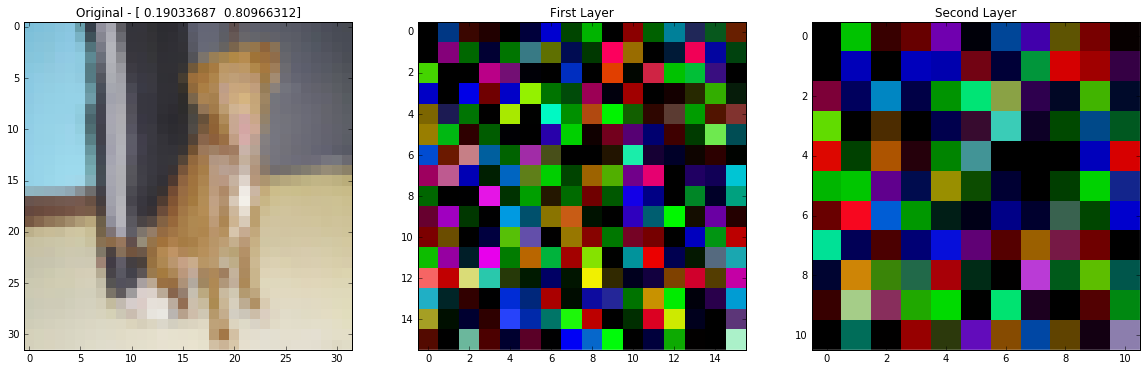In :
def layers_output(idx):
input_image = testData[idx]
first_output = input_layer_out.predict(input_image.reshape(1,testData[idx].shape))
second_output = hidden_layer_out.predict(input_image.reshape(1,testData[idx].shape))
second_output.resize(11*11*3)
final_output =  model.predict(input_image.reshape(1,input_image.shape))
return input_image,first_output,second_output,final_output

total_imgs = 10

plt.figure(figsize=(10,30))
for i in range(total_imgs):
input_image,first_output,second_output,final_output = layers_output(i)
plt.subplot(total_imgs,3,1+i*3)
plt.imshow((input_image.reshape(32,32,3)), interpolation='none')
plt.title("Original - "+str(final_output))
plt.subplot(total_imgs,3,2+i*3)
plt.imshow(first_output.reshape(16,16,3),interpolation='none')
plt.title("First Layer")
plt.subplot(total_imgs,3,3+i*3)
plt.imshow(second_output.reshape(11,11,3),interpolation='none')
plt.title("Second Layer")
plt.tight_layout()
plt.show()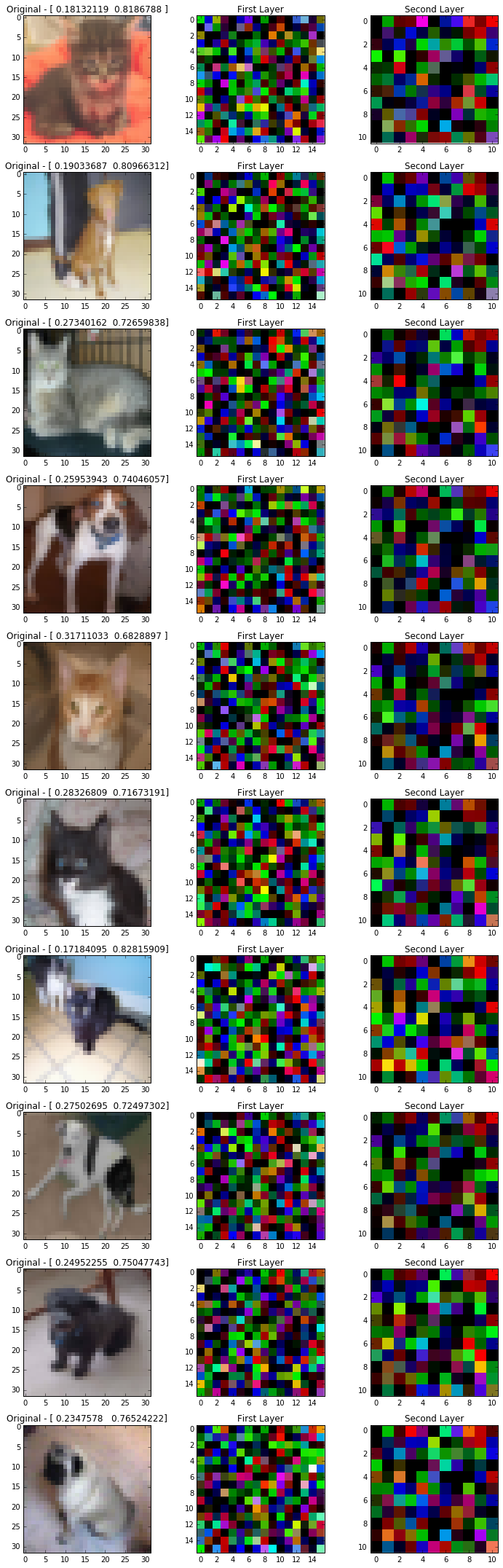### 2. Recover the weights and load into the new network¶

All layers (??? at least the ones I've tested so far...) have two methods to get and set weights:

• get_weights
• set_weights
In :
w1 = first_layer.get_weights()
w2 = second_layer.get_weights()
w3 = classifier_layer.get_weights()

In :
# Dense layers fully connect the input to the output (w1) and add bias (w1).
# Therefore get_weights returns the weights matrix and the bias vector
# (actually bias can be seen as always 1 and the values are the weights connecting them to the next layer, one-to-one).
w1.shape, w1.shape

Out: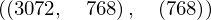I hope you can do it from here ;)

In :
w1.max()

Out:
0.096393012
In :
w1.min()

Out:
-0.097316824
In :
w1_reshaped = w1.reshape((32,32,3,768))

In :
w1_reshaped[:,:,0].flatten()

Out:
array([-0.03662795, -0.00825124, -0.01432665, ..., -0.01016488,
-0.01302101, -0.02127896], dtype=float32)

As a test, I will load the weights from the model 'my_97perc_acc' into the 'model_rnd'

In :
# First instanciate the layer:
first_layer_rnd = model_rnd.get_layer('Input_layer')
# we could also use model_rnd.layers instead

second_layer_rnd = model_rnd.get_layer('Hidden_layer')

classifier_layer_rnd = model_rnd.get_layer('Classifier_layer')

In :
# just saves the current weights
w1_old = first_layer_rnd.get_weights()
w2_old = second_layer_rnd.get_weights()
w3_old = classifier_layer_rnd.get_weights()

In :
first_layer_rnd.set_weights(w1_old)
second_layer_rnd.set_weights(w2_old)
classifier_layer_rnd.set_weights(w3_old)

idx = 1
input_image = testData[idx]
first_output = input_layer_out.predict(input_image.reshape(1,testData[idx].shape))
second_output = hidden_layer_out.predict(input_image.reshape(1,testData[idx].shape))
second_output.resize(11*11*3)
final_output =  model.predict(input_image.reshape(1,input_image.shape))

plt.figure(figsize=(20,10))
plt.subplot(131)
plt.imshow((input_image.reshape(32,32,3)), interpolation='none')
plt.title("Original - "+str(final_output))
plt.subplot(132)
plt.imshow(first_output.reshape(16,16,3),interpolation='none')
plt.title("First Layer")
plt.subplot(133)
plt.imshow(second_output.reshape(11,11,3),interpolation='none')
plt.title("Second Layer")
plt.show()In :
# set the weights using the ones from our trained model:
# (the results must be the same now...)
first_layer_rnd.set_weights(w1)
second_layer_rnd.set_weights(w2)
classifier_layer_rnd.set_weights(w3)

idx = 1
input_image = testData[idx]
first_output = input_layer_out.predict(input_image.reshape(1,testData[idx].shape))
second_output = hidden_layer_out.predict(input_image.reshape(1,testData[idx].shape))
second_output.resize(11*11*3)
final_output =  model.predict(input_image.reshape(1,input_image.shape))

plt.figure(figsize=(20,10))
plt.subplot(131)
plt.imshow((input_image.reshape(32,32,3)), interpolation='none')
plt.title("Original - "+str(final_output))
plt.subplot(132)
plt.imshow(first_output.reshape(16,16,3),interpolation='none')
plt.title("First Layer")
plt.subplot(133)
plt.imshow(second_output.reshape(11,11,3),interpolation='none')
plt.title("Second Layer")
plt.show()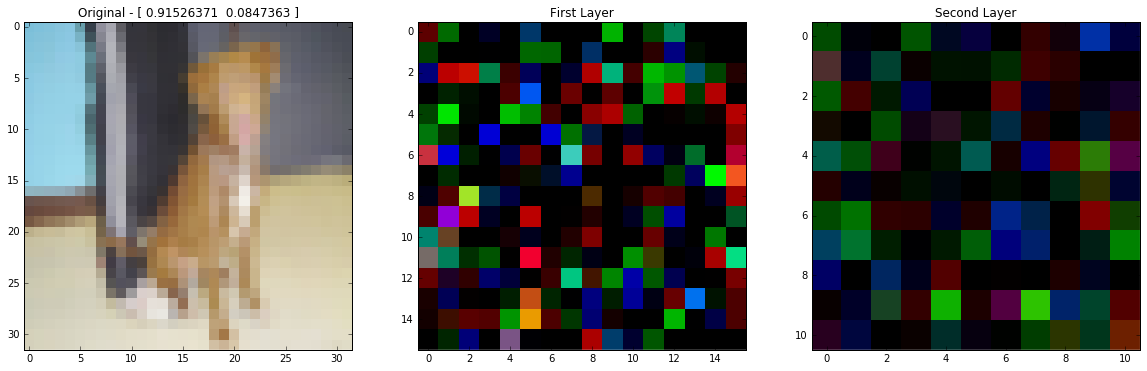In [ ]: